Type 3 Analysis

A Type 3 analysis is similar to the Type III sums of squares used in PROC GLM, except that likelihood ratios are used instead of sums of squares. First, a Type III estimable function is defined for an effect of interest in exactly the same way as in PROC GLM. Then maximum likelihood estimates are computed under the constraint that the Type III function of the parameters is equal to 0, by using constrained optimization. Let the resulting constrained parameter estimates be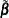and the log likelihood be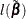. Then the likelihood ratio statistic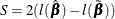where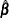is the unconstrained estimate, has an asymptotic chi-square distribution under the hypothesis that the Type III contrast is equal to 0, with degrees of freedom equal to the number of parameters associated with the effect.

When a Type 3 analysis is requested, PROC GENMOD produces a table that contains the likelihood ratio statistics, degrees of freedom, and p-values based on the limiting chi-square distributions for each effect in the model. If you specify either the DSCALE or PSCALE option in the MODEL statement, F statistics are also computed for each effect.

Options for handling the dispersion parameter are the same as for a Type 1 analysis. The dispersion parameter can be specified to be a known value, estimated from the deviance or Pearson’s chi-square divided by degrees of freedom, or estimated by maximum likelihood individually for the unconstrained and constrained models. By default, PROC GENMOD estimates scale by maximum likelihood for each model fit.

The results of this type of analysis do not depend on the order in which the terms are specified in the MODEL statement.

A Type 3 analysis can consume considerable computation time since a constrained model is fitted for each effect. Wald statistics for Type 3 contrasts are computed if you specify the WALD option. Wald statistics for contrasts use less computation time than likelihood ratio statistics but might be less accurate indicators of the significance of the effect of interest. The Wald statistic for testing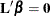, whereis the contrast matrix, is defined by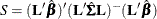whereis the maximum likelihood estimate and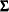is its estimated covariance matrix. The asymptotic distribution of S is chi-square with r degrees of freedom, where r is the rank of.

See Chapter 42: The GLM Procedure,and Chapter 15: The Four Types of Estimable Functions, for more information about Type III estimable functions. Also see Littell, Freund, and Spector (1991).

Generalized score tests for Type III contrasts are computed for GEE models if you specify the TYPE3 option in the MODEL statement when a REPEATED statement is also used. See the section Generalized Score Statistics for more information about generalized score statistics. Wald tests are also available with the Wald option in the CONTRAST statement. In this case, the robust covariance matrix estimate is used forin the Wald statistic.